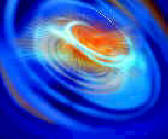#Wordtrade.com Mathematics

### Review Essays of Academic, Professional & Technical Books in the Humanities & Sciences

Perturbation Techniques in Mathematics, Engineering & Physics by Richard Ernest Bellman (Dover) unabridged republication of the edition originally published by Holt, Rinehart and Winston, New York, 1966. Indexes. 7 figures.

An introduction to a variety of perturbation techniques for ordinary differential equations, this work outlines applications through specific examples. Mathematicians, engineers, and applied scientists will find its exposition entirely accessible.

The first part covers the classical perturbation techniques. Dr. Bellman begins with a readily solved linear algebraic equation that exhibits some of the versatility of the Lagrange expansion theorem. This leads by easy stages to a discussion of the matrix exponential and to a brief excursion into the domain of Poincaré and Lyapunov, where invariant imbedding is illustrated. Subsequent discussions of alternative perturbation techniques employ dynamic programming. The second chapter concentrates on nonlinear differential equations, presenting renormalization techniques of Lindstedt and Shohat and averaging techniques by Bellman and Richardson. The concluding chapter considers a variety of questions centering on a second-order linear differential equation, explaining the applications of the WKB-Liouville method and asymptotic series.

Exercises, comments, and an annotated bibliography follow each demonstration of technique. The text presupposes a course in intermediate calculus and rudiments of the theory of ordinary differential equations. For the engineer, physicist, or mathematician working in fields requiring the solution of differential equations, this text provides a stimulating introduction to analytical approximation techniques.